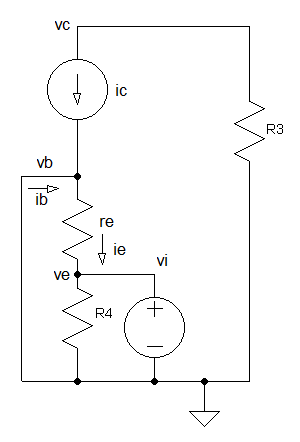# BJT Common Base Amplifier

A common base amplifier is one of three basic single-stage bipolar junction transistor (BJT) amplifier configuration, typically used as a current buffer or voltage amplifier. In this configuration, the emitter terminal of the transistor serves as the input, the collector the output, and the base is common and connected to ground (through Cb).This circuit is usually found in high-frequency amplifiers because its input capacitance does not suffer from the Miller effect, which degrades the bandwidth of the common emitter configuration, and because of the relatively high isolation between the input and output.

It is also used as current buffer since it has a current gain of approximately unity. When the circuit is preceded by a common emitter stage, it is called a cascode circuit. The cascode circuit has the benefits of both configurations, such as high input impedance and isolation.

## DC Analysis

First we redraw the schematic using the BJT DC model. Capacitors are considered open circuit in DC and therefore are excluded.IB can be ignored if \begin{equation} 10R_2 < \beta R_4 \end{equation} Thus VB can be calculated using KVL as simple voltage divider circuit \begin{equation} V_B = {R_2 \over {R_1 + R_2}} V_S \end{equation} Current at Node E \begin{equation} I_E = I_B + I_C \end{equation} if IC is much greater than IB, IB can be ignored \begin{equation} I_E = I_C \end{equation}

Using KVL (Kirchhoff's voltage law) \begin{equation} V_B = I_ER_4 + V_{BE} \end{equation} \begin{equation} V_S = I_CR_3 + V_{C} \end{equation}

To obtain maximum output swing, you should choose the resistor values such that VC is half the supply voltage.

\begin{equation} V_{C} = {V_S \over 2} \end{equation}

## AC Analysis

Next we redraw the schematic using the BJT small signal model. Capacitors are considered shorts in AC (R4 is shorted out by Ce) and DC supplies are connected to GND (ground). Calculate re

\begin{equation} r_e = {v_T \over I_E} \end{equation}Since the input voltage vi is across re and using ohm's law \begin{equation} i_e = -{v_i \over r_e} \end{equation} the negative sign is due to direction of ie.

The output voltage is \begin{equation} v_c = -i_cR_3 \end{equation} the inverted output is due to the current direction.

From KCL we know that \begin{equation} i_e = i_b + i_c \end{equation} By ignoring ib from the equation since it is small compared to ic, we obtain \begin{equation} v_c = -i_eR_3 \end{equation}

Applying equation 9 to equation 12, the voltage gain of the amplifier is \begin{equation} {v_c \over v_i} = {R_3 \over r_e} \end{equation}

Current through R4 due to Vi can be ignored if

\begin{equation} R_4 > 10 r_e \end{equation}

Since we can ignore ib, then by inspection the current gain is \begin{equation} {i_c \over i_e} = 1 \end{equation}

With advertising revenues falling despite increasing numbers of visitors, we need your help to maintain and improve this site, which takes time, money and hard work. Thanks to the generosity of our visitors who gave earlier, you are able to use this site at no charge.

If you have benefited from this site and are able, please give \$10 via Paypal. It will allow us continue into the future. It only takes a minute. Thanks!